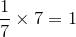Search IntMath
Close

450+ Math Lessons written by Math Professors and Teachers

5 Million+ Students Helped Each Year

1200+ Articles Written by Math Educators and Enthusiasts

Simplifying and Teaching Math for Over 23 Years

# Math movie: How to play a Rubik’s Cube like a piano

By Murray Bourne, 09 Dec 2015

We use group theory every day, without ever thinking about it. Here's a simple example.

The integers form a group under the operation of addition.

4 + − 4 = 0

In this case, 0 is the identity and − 4 is the inverse. This example also demonstrates closure (4 and − 4 are integers, and when we add them, we also get an integer, 0.)

Another property of a group is that it is associative. That is, if we have more than one operation, it doesn't matter what order we do it in. For example:

(4 + − 4) + 3 = 4 + (− 4 + 3) = 3

Similarly, the positive rational numbers form a group under multiplication. If we start with the number 7, we can find its inverse, 1/7 and the identity is 1. Thus we have:Similarly, each of 1/7, 7 and 1 are rational numbers, so the group is closed.

## Many kinds of groups

Group theory covers many different areas, including permutations, matrices, transformations, topology and so on.

Applications include number theory, chemistry, physics, mechanics and music.

## Group theory and music

Notes on a piano are periodic. That is, the 12 notes starting from the lower end of the piano are repeated 7 times to form the rest of the keyboard.

A, A#, B, C, C#, D, D#, E, F, F#, G, G#

A lot of music makes use of what is called the cycle of 5ths, where the chord structure follows notes which are spaced 5 notes apart, like this:

B E A D G C F A# D# G# C# F#

This forms a cyclic group structure which can also be periodic.

And this brings me to today's math movie. It's a TED-Ed offering by Michael Staff and outlines the connections between Rubik's Cube and music.

Be the first to comment below.

### Comment Preview

HTML: You can use simple tags like <b>, <a href="...">, etc.

To enter math, you can can either:

1. Use simple calculator-like input in the following format (surround your math in backticks, or qq on tablet or phone):
a^2 = sqrt(b^2 + c^2)
(See more on ASCIIMath syntax); or
2. Use simple LaTeX in the following format. Surround your math with $$ and $$.
$$\int g dx = \sqrt{\frac{a}{b}}$$
(This is standard simple LaTeX.)

NOTE: You can mix both types of math entry in your comment.